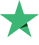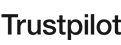65 学生
121 课

#### 您将学到什么?

• You'll know how and why use R for data science and machine learning
• You'll get practical tips and advise on Data Scientist's career
• You'll work on practical tasks and examine case studies to fully understand the fundamentals of R programming
• You'll be able to use the core features and packages of R

#### 教学大纲

22点 31分
02:40
##### 2: Introduction to Data Science 部分
56:59
Introduction To Data Scientist
12:31
How to switch your career into ML part 1
14:56
How to switch your career into ML part 2
03:31
##### 3: Course Curriculum Overview 部分
22:41
Course Curriculum Overview
22:41
##### 4: Introduction to R 部分
26:15
Introduction to R
15:07
Setting up R
11:08
##### 5: R Programming 部分
55:14
R Operator
13:45
R Conditional Statement & Loop
12:02
R Programming - R Function #1
13:20
R Programming - R Function #2
10:10
R Programming - R Function #3
05:57
##### 6: R Data Structure 部分
49:23
An Introduction of R Data Structure + Vector
11:13
Matrix, Array and Data Frame
14:37
A Deep Drive to R Data Frame
13:02
Factor
04:12
R Data Structure - List
06:19
##### 7: Import and Export in R 部分
32:16
Import CSV Data in R
09:26
Import Text Data in R
03:19
Import Excel, Web Data in R
12:47
Export Data in R - Text
02:37
Export Data in R - CSV & Excel
04:07
##### 8: Data Manipulation 部分
1:33:49
Data Manipulation - Apply Function
13:15
Data Manipulation - select
11:46
Data Manipulation - mutate
14:28
Data Manipulation - filter
14:11
Data Manipulation - arrange
09:38
Data Manipulation - Pipe Operator
08:30
Data Manipulation - group by
11:26
Data Manipulation - Date
10:35
##### 9: Data Visualization 部分
2:10:04
Introduction to Data Visualiztion & Scatter Plot
12:01
Data Visualization - mfrow
07:37
Data Visualization - pch
12:30
Data Visualization - Color
01:19
Data Visualization - Line Chart
03:21
Data Visualization - Bar Plot
07:05
Data Visualization - Pie Chart
06:43
Data Visualization - Histogram
07:06
Data Visualization - Density Plot
02:26
Data Visualization - Box Plot
05:01
Data Visualization - Mosaic Plot and Heat Map
07:59
Data Visualization - 3D Plot
10:39
Correlation Plot and Word Cloud
09:02
Data Visualization - ggplot2 Part 1
14:03
Data Visualization - ggplot2 Part 2
08:08
Data Visualization - ggplot2 Part 3
15:04
##### 10: Introduction To Statistics 部分
2:02:57
Introduction To Statistics Part 1
13:25
Introduction To Statistics Part 2
08:53
Introduction To Statistics Part 3
14:55
Introduction To Statistics Part 4
04:15
Introduction To Statistics Part 5
15:10
Introduction To Statistics Part 6
08:21
Introduction To Statistics Part 7
15:04
Introduction To Statistics Part 8
10:45
Introduction To Statistics Part 9
10:24
Introduction To Statistics Part 10
14:34
Introduction To Statistics Part 11
07:11
##### 11: Hypothesis Testing 部分
41:58
Hypothesis Testing Part 1
10:08
Hypothesis Testing Part 2
11:28
Hypothesis Testing Part 3
14:21
Hypothesis Testing Part 4
06:01
##### 12: Hypothesis Testing in Practice 部分
2:14:01
Hypothesis Testing in Practice Part 1
15:04
Hypothesis Testing in Practice Part 2
09:36
Hypothesis Testing in Practice Part 3
14:16
Hypothesis Testing in Practice Part 4
12:36
Hypothesis Testing in Practice Part 5
10:29
Hypothesis Testing in Practice Part 6
13:46
Chi Square Part 1
11:19
Chi Square Part 2
14:57
ANOVA Part 1
12:30
ANOVA Part 2
14:20
What we discussed in the chapter so far?
05:08
##### 13: Machine Learning Toolbox 部分
26:31
Machine Learning Toolbox Part 1
14:00
Machine Learning Toolbox Part 2
12:31
12:51
12:51
##### 15: Data Pre-Processing 部分
1:24:14
Data Pre-Processing part 1
14:45
Data Pre-Processing part 2
14:29
Data Pre-Processing part 3
10:25
Data Pre-Processing part 4
09:39
Data Pre-Processing part 5
12:33
Data Pre-Processing part 6
07:19
Data Pre-Processing part 7
15:04
##### 16: Supervised Learning : Regression 部分
3:06:14
Linear Regression part 1
11:47
Linear Regression part 2
14:23
Linear Regression part 3
20:21
Linear Regression part 4
19:02
Linear Regression part 5
25:00
Linear Regression part 6
15:02
Linear Regression part 7 - Correlation Part 1
14:31
Linear Regression part 7 - Correlation Part 2
13:44
Linear Regression part 8 - Stepwise Regression
12:52
Linear Regression part 9 - Stepwise Regression
16:03
Linear Regression part 10 - Dummy Variable
12:34
Linear Regression part 11 - Non Linear
10:55
##### 17: Classification Overview 部分
13:29
Classification Overview
13:29
##### 18: Logistic Regression 部分
1:11:26
Logistics Regression Intuition
14:04
R Code Implementation Part 1
05:09
R Code Implementation Part 2
10:37
Model Evaluation
12:28
Telecom Churn Case Study
22:27
Summary
06:41
##### 19: K-NN Study 部分
40:13
K-NN Intuition
13:26
K-NN R Code Implementation
12:48
K-NN Case Study
13:59
##### 20: SVM Study 部分
45:18
SVM - Intuition
08:44
SVM - R Code Implementation
08:22
SVM - Model Tuning
09:00
SVM - Telecom Case Study
07:56
SVM - Non Separable Case and Pros and Cons
07:27
SVM Chapter summary
03:49
##### 21: Naive Bayes 部分
36:46
Naive Bayes - Intuition
19:56
Naive Bayes - R Code Implementation
08:25
Naive Bayes - Case Study
08:25
##### 22: Decision Tree 部分
1:06:18
Decision Tree Intuition
14:54
Decision Tree - How it works
07:40
Decision Tree - R Code Implementation
13:44
Decision Tree - Pruning
15:36
Decision Tree - Case Study
14:24

#### 要求

• No prior knowledge is required to enrol this course
• Software and data needed in the course will be provided for free

#### BitDegree平台评价

9.5 于 10